Fabina borrows Rs 12,500 at 12% per annum for 3 years at simple interest and Radha borrows the same amount for the same time period at 10% per annum, compounded annually. Who pays more interest and by how much?

You can also refer to the link:

https://img-nm.mnimgs.com/img/study_content/curriculum_videos/1/8/1/8/134/V-8-1-8-P134-S1-Q3-3233-1.swf

• 3Interest paid by Fabina is Rs. 362.50 more than that paid by radha.

• 8

SI = Principal*rate*time/100

= 12500*12*3/100

= 1500*3

= 4500

Amount = Principal + SI

= 12500+4500

= 17000

Therefore Fabina paid Rs.17000

Amount Of CI = Principal(1+Rate/100)Time

=12500(1+12/100)3

= 12500(112/100)3

= 12500(1.4)

= 12500*1.4

=17561

• -1

Ans. Here, Principal (P) = Rs.12,500, Time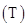= 3 years, Rate of interest (R)

= 12% p.a.

Simple Interest for Fabina =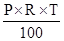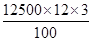= Rs. 4,500

Amount for Radha, P = Rs. 12,500, R = 10% and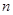= 3 years

Amount (A)  =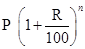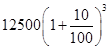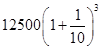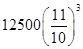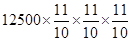= Rs. 16,637.50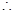C.I. for Radha = A – P

= Rs. 16,637.50 – Rs. 12,500 = Rs. 4,137.50

Here, Fabina pays more interest

= Rs. 4,500 – Rs. 4,137.50 = Rs. 362.50

• 0
What are you looking for?